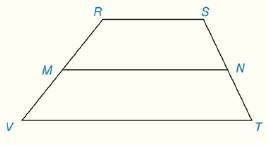Chapter 4.CT, Problem 14CTElementary Geometry For College St...

7th Edition
Alexander + 2 others
ISBN: 9781337614085

Solutions

Chapter
SectionElementary Geometry For College St...

7th Edition
Alexander + 2 others
ISBN: 9781337614085
Textbook Problem

In trapezoid RSTV of Exercise 13, R S ¯ ∥ V T ¯ and M N ¯ is the median, Find x if V T = 2 x + 9 , M N = 6 x − 13 ,  and  R S = 15 .Exercise 13, 14

To determine

To Find:

The value of x.

Explanation

Given:

Trapezoid RSTV, RS¯VT¯ and MN¯ is the median.

VT=2x+9,MN=6x13, and RS=15.

Calculation:

The length of the median of a trapezoid equals one-half the sum of the lengths of two bases

RS¯ and VT¯ are the bases of the trapezoid.

Still sussing out bartleby?

Check out a sample textbook solution.

See a sample solution

The Solution to Your Study Problems

Bartleby provides explanations to thousands of textbook problems written by our experts, many with advanced degrees!

Get Started

Solve the equations in Exercises 126. 14x2=0

Finite Mathematics and Applied Calculus (MindTap Course List)

If f is continuous and 02f(x)dx=6, evaluate 0/2f(2sin)cosd.

Single Variable Calculus: Early Transcendentals, Volume I

Evaluate the integral. 70. 11+2exexdx

Single Variable Calculus: Early Transcendentals